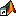## 在 Windows 平台上启动 MATLAB

MATLAB 启动文件夹是您在获得 MATLAB 提示符时所在的文件夹。要更改默认启动文件夹，请参阅 MATLAB 启动文件夹

### 选择 MATLAB 图标

• 您的 Windows 桌面上的安装程序快捷方式 - 双击 MATLAB 图标• Windows 10 系统 - 开始 > 所有应用 > MATLAB `Release`

• Windows 8 系统 - 在“开始”屏幕或桌面上，点击 MATLAB `Release`

• Windows 7 系统 - 如果选择让安装程序在 Windows 的“开始”菜单中放置 MATLAB 程序的快捷方式，则选择开始 > MATLAB `Release`

1. 右键点击 MATLAB 的快捷方式图标，然后从上下文菜单中选择属性。MATLAB 的“属性”对话框将在快捷方式窗格中打开。

2. 启动位置字段中，键入系统上某个文件夹的完整路径，然后点击

### 从 Windows 系统命令行调用 `matlab`

`matlab -useStartupFolderPref`

### 从 MATLAB 命令提示符调用 `matlab`

`!matlab`

### 打开与 MATLAB 相关联的文件

MATLAB 安装程序会设置某些文件类型与 MathWorks® 产品之间的关联。在您打开此类文件之一时将启动 MATLAB。启动文件夹是包含该文件的文件夹。

### 从 Windows 资源管理器工具中选择 MATLAB 可执行文件

• 上一个 MATLAB 会话的最后一个工作文件夹。

• 初始工作文件夹预设项中指定的自定义路径。